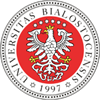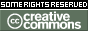REPOZYTORIUM UNIWERSYTETU
W BIAŁYMSTOKUProszę używać tego identyfikatora do cytowań lub wstaw link do tej pozycji: `http://hdl.handle.net/11320/8128`
 Tytuł: Formalization of the MRDP Theorem in the Mizar System Autorzy: Pąk, Karol Słowa kluczowe: Hilbert’s 10th problemDiophantine relations11D4568T9903B35 Data wydania: 2019 Data dodania: 29-lip-2019 Wydawca: DeGruyter Open Źródło: Formalized Mathematics, Volume 27, Issue 2, Pages 209 - 221 Abstrakt: This article is the final step of our attempts to formalize the negative solution of Hilbert’s tenth problem.In our approach, we work with the Pell’s Equation defined in . We analyzed this equation in the general case to show its solvability as well as the cardinality and shape of all possible solutions. Then we focus on a special case of the equation, which has the form x2 − (a2 − 1)y2 = 1  and its solutions considered as two sequences {xi(a)}i=0∞,{yi(a)}i=0∞. We showed in  that the n-th element of these sequences can be obtained from lists of several basic Diophantine relations as linear equations, finite products, congruences and inequalities, or more precisely that the equation x = yi(a) is Diophantine. Following the post-Matiyasevich results we show that the equality determined by the value of the power function y = xz is Diophantine, and analogously property in cases of the binomial coe cient, factorial and several product .In this article, we combine analyzed so far Diophantine relation using conjunctions, alternatives as well as substitution to prove the bounded quantifier theorem. Based on this theorem we prove MDPR-theorem that every recursively enumerable set is Diophantine, where recursively enumerable sets have been defined by the Martin Davis normal form.The formalization by means of Mizar system , ,  follows , Z. Adamowicz, P. Zbierski  as well as M. Davis . Afiliacja: Institute of Informatics, University of Białystok, Poland URI: http://hdl.handle.net/11320/8128 DOI: 10.2478/forma-2019-0020 ISSN: 1426-2630 e-ISSN: 1898-9934 metadata.dc.identifier.orcid: 0000-0002-7099-1669 Typ Dokumentu: Article Występuje w kolekcji(ach): Artykuły naukowe (WMiI)Formalized Mathematics, 2019, Volume 27, Issue 2

Pliki w tej pozycji:
Plik Opis RozmiarFormat
Pozycja ta dostępna jest na podstawie licencji Licencja Creative Commons CCL